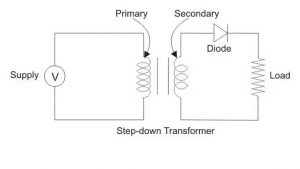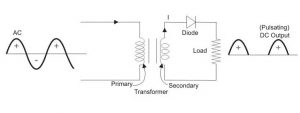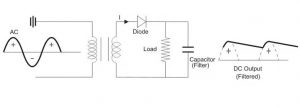# Complete Guide for Half Wave Rectifier

A rectifier is a device that converts alternating current (AC) to direct current (DC) using one or more P-N junction diodes (DC). Half-wave rectifiers convert AC electricity to DC voltage. A single diode performs the transition in a half-wave rectifier circuit. In a half-wave rectifier, an AC voltage waveform can only travel in a one-half cycle of the input voltage and blocks the other half.

## What is a Half Wave Rectifier?

A half-wave rectifier is a diode circuit that converts alternating current (AC supply) to direct current (DC Supply). In the HWR circuit, a single diode is utilized to convert AC to DC.## Half Wave Rectifier Circuit Diagram

The circuit of Half Wave Rectifier consists of three main components:

• A Diode
• A Transformer
• A Load (may be a Resistance)## Half Wave Rectifier Waveform

A half-wave rectifier is the simplest basic form of the rectifier, requiring only one diode to be placed into a circuit. A half-wave rectifier circuit exposes a single diode in series with a load resistor to an alternating current.## Working of Half Wave Rectifier

### Positive Half-Cycle

The AC high voltage is provided to the step-down transformer’s primary, and the resultant secondary low voltage is applied to the diode, which is forward biassed during the positive half cycle and acts as a short circuit.### Negative Half Cycle

When the P – terminal of the diode is connected to the negative supply during the negative half cycle, the diode operates like an open circuit and does not create the output across the load.

## Half Wave Rectifier Formulae

These are some characteristics of the half-wave rectifier

##### Half Wave Rectifier Efficiency

HWR efficiency is defined as the ratio of output DC power to input AC power.

$$\eta = \frac{ P_{dc} }{ P_{ac} }$$

Half-wave rectifiers have a maximum efficiency of 40.6%.

##### Half Wave Rectifier Ripple Factor

The ripple factor of a halfwave rectifier defines how successfully it can convert AC to DC current.

The following formula is used to calculate the ripple factor:

$$\gamma = \sqrt{ ( \frac{ V_{rms} }{ V_{dc} } )^{2}-1 }$$

A halfwave rectifier has a ripple factor of 1.21.

##### Half Wave Rectifier RMS value

For a half-wave rectifier, the RMS value of the load current is given by the formula:

$$RMS= \frac{Max. Value}{2}$$

$$V_{RMS} = \frac{ V_{m} }{2}$$

##### Halfwave Rectifier Form Factor

The form factor is the ratio of the RMS value to the average value, as calculated by the formula:

Form Factor = RMS Value / Avg. Value

Form Factor= $$\frac{ V_{rms} }{ V_{avg} }$$

## Half wave rectifier with Capacitor Filter

A halfwave rectifier’s output waveform is a pulsing DC waveform. Filters in halfwave rectifiers convert pulsing waveforms to continuous DC waveforms. As a filter, a capacitor or an inductor can be employed.

The circuit schematic below demonstrates how a capacitive filter is used in conjunction with a halfwave rectifier to smooth out a pulsing DC waveform and convert it to a continuous DC waveform.### Pros and Cons of Half Wave Rectifier

##### Advantages of a half-wave rectifier
• A simple Circuit
• It is affordable
• It is simple to use
• It is easy to build
• It is inexpensive since it has a small number of components
##### Disadvantages of a half-wave rectifier
• The usage factor of the transformer is poor
• They generate a low-output voltage
• DC saturation of the transformer core causes magnetizing current, hysteresis losses, and harmonic production
• Power output and hence rectification efficiency are both extremely poor. This is because electricity is only given during the one-half cycle of the input alternating voltage
• Because the ripple factor is significant, sophisticated filtering is necessary to provide a consistent dc output
• They only let a half-cycle of each sinewave get through, and the other half-cycle is squandered. This results in a loss of power

## Conclusion

Learn more about Half Wave Rectifier with Tutoroot’s real online interactive classes. We have expert faculty who are alumni of IITs to help you in your learning journey. Take a look at the courses offered by tutoroot.

## FAQ’s

What is the peak inverse voltage of half wave rectifier?

Vm is the peak inverse voltage of the diode utilized in the half-wave rectifier.

What is the RMS value of the half-wave Rectifier?

A half-wave rectifier current has an RMS value of 10 Ampere.

What is the Ripple Factor of Half Wave Rectifier?

A half-wave rectifier has a ripple factor of 1.21.